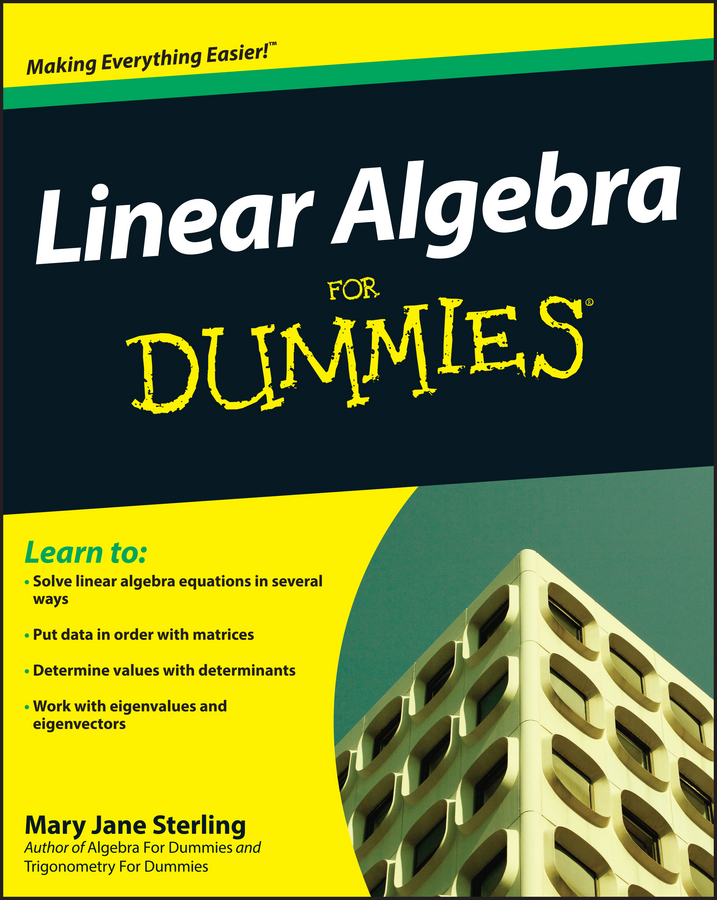##### Linear Algebra For DummiesThe associative property comes in handy when you work with algebraic expressions. Use the associative property to change the grouping in an algebraic expression to make the work tidier or more convenient. Just keep in mind that you can use the associative property with addition and multiplication operations, but not subtraction or division, except in a few special cases.

Think about what the word associate means. When you associate with someone, you’re close to the person, or you form a group with the person. Say that Anika, Becky, and Cora associate. Whether Anika drives over to pick up Becky and the two of them go to Cora’s and pick her up, or Cora is at Becky’s house and Anika picks up both of them at the same time, the same result occurs — the same people are in the car at the end.

Check out how the associative property works in the following examples:

• Addition: a + (b + c) = (a + b) + c

4 + (5 + 8) = 4 + 13 = 17, and (4 + 5) + 8 = 9 + 8 = 17

You can group the numbers however you want to and still reach the same result, 17.

• Multiplication: a × (b × c) = (a × b) × c

3 × (2 × 5) = 3 × 10 = 30, and (3 × 2) × 5 = 6 × 5 = 30

This example shows you two options for grouping the numbers — but the result, 30, is the same regardless of how you group the numbers.

• Subtraction: a – (bc) ≠ (ab) – c (except in a few special cases)

13 – (8 – 2) = 13 – 6 = 7, but (13 – 8) – 2 = 5 – 2 = 3

Now you can see how subtraction doesn't follow the associative property. Regrouping the numbers resulted in two different answers.

• Division: a ÷ (b ÷ c) ≠ (a ÷ b) ÷ c (except in a few special cases)

48 ÷ (16 ÷ 2) = 48 ÷ 8 = 6, but (48 ÷ 16) ÷ 2 = 3 ÷ 2 = 1.5

This example illustrates how division doesn't follow the associative property. Regrouping the numbers resulted in two different answers.

You can always find a few cases where the property works even though it isn’t supposed to. For instance, in the subtraction problem 5 – (4 – 0) = (5 – 4) – 0 the property seems to work. Also, in the division problem
6 ÷ (3 ÷ 1) = (6 ÷ 3) ÷ 1, it seems to work.